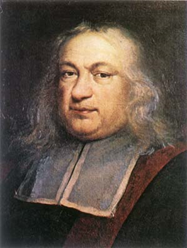# More on Diophantus and Fermat

Posted by Graham Wheeler on Sunday, January 3, 2010

In a previous post I wrote about how Fermat scribbled his famous “last theorem” in the margin of Diophantus’ Arithmetica. This is called Fermat’s last theorem not because it was the last thing Fermat wrote but because of all the incomplete theorem’s we know were left by Fermat it was the last to be proved, taking about 350 years.

The section of the book where Fermat wrote his comment was on finding Pythagorean triples: square numbers whose sums also form squares. Such numbers can be the sides of Pythagorean (right-angled) triangles, the most well know being 3-4-5.

The Arithmetica was a book of computational methods rather than theoretical mathematics. In modern terms we would call it a book of numerical algorithms. Much of the material was not invented by Diophantus but was collected by him. This includes his method for finding Pythagorean triples, which was as follows. Take any two whole numbers $$a$$ and $$b$$. Then the following three numbers are the sides of a Pythagorean triangle:

• $$2ab$$
• $$a^2 - b^2$$
• $$a^2 + b^2$$

Furthermore, any such triple can be multiplied by a constant to get another triple (e.g. the triple 3-4-5 can be multiplied by 3 to get the triple 9-12-15, etc).Fermat’s theorem asserts that while there are an infinite number of Pythagorean triples, there are no solutions in whole numbers for higher powers. Fermat did leave a proof for the fourth power. Euler proved the theorem for the third power. Dirichlet proved the theorem for fifth and 14th powers. Lame and Kummer managed to prove the theorem for all powers up to 100 except for 37, 59 and 67. By 1980, the theorem had been proven for all powers up to 125,000!

It took some modern developments in elliptic functions to proceed further. Elliptic functions are functions in two unknowns where one unknown is squared and the other cubed (e.g. $$y^2 = x^3 - x - 1$$). It was found that if Fermat was wrong, and there is a solution for some power higher than two, then that solution describes a very unusual elliptic curve, so unusual it seems unlikely it could exist.

In 1986 Kenneth Ribet showed that such an elliptic curve would violate the 1955 Taniyama-Shimura conjecture, which claims that every elliptic curve is associated with a modular function. While the Taniyama-Shimura conjecture was not itself proven this did provide a link between two fields of mathematics, and it was this conjecture that was ultimately proven by Andrew Wiles and a former student in 1993 and 1994, thus finally proving Fermat correct.

If Fermat really did have a proof, though, then there is a much simpler proof still waiting to be found…